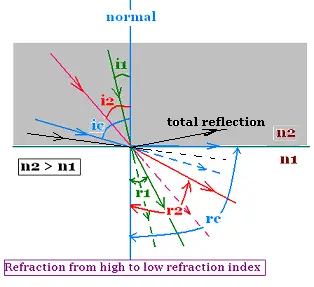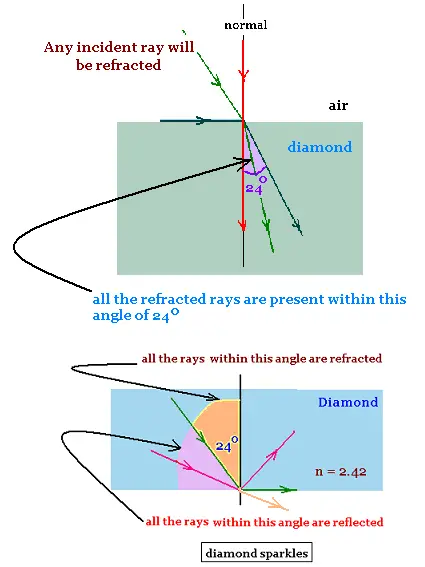Optics -     Geometrical Optics..

### 1. Critical angle: total reflectionThe ray propagates from a medium of high refraction index n2 to a medium of lower refraction index n1 (n2 > n1).

The incident ray (incidence angle i1) has its related refracted ray (refraction angle r1). The incident ray (incidence angle i2) has its related refracted ray (refraction angle r2). The incident ray (incidence angle ic) has its related refracted ray (refraction angle rc). Any other ray of incidence angle bigger that ic has no refracted ray and it is totally reflected. The angle ic is the critical angle for wich the refracted angle is right (π/2).

Let's write n2 sin ic = n1 sin (π/2) = n1. Then:
sin ic = n1 /n2; so ic = arcsin(n1/n2)

The critical angle is given by:

ic = arcsin(n1/n2)

### 2. Example:If the medium 2 is diamond, we will have:
n2 = 2.42. For n1 (air) = 1, then:
ic = arcsin(n1/n2) = arcsin(1/2.42) → ic = 24.41 o.
The critical angle for diamond is 24.41 o
That is:
r = arcsin(2.42 sin i)
i = 5o → r = 12.20 o.
i = 24.41o → r = 90 o.
i = 35o → r : = invalid .
All the rays within the angle of 24o, are refracted, and all the rays within the angle of 90o - 24o = 66o, are reflected. The diamond as substance keeps much of the light rays. This is what makes it sparkle, when exposed to light.